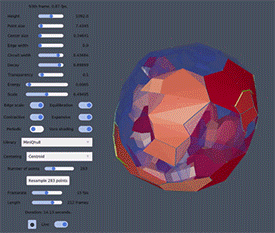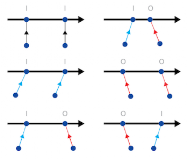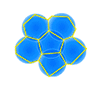## Monday, December 20, 2021

### Voro-XThe past months I have been in contact with MIT postdoc Benoît Legat a mathematician in numerical geometry, who has converted the 2D DynamicFoam model, developed by an other MIT affiliate Nick Weigert, into a 3D application:

Voro-X

The name stands for Voronoi diagrams (foam) where energetic currents run through the edges of the mesh, and X-ings (junctions) that act like a gates where currents are switched OFF or ON based on the sharpness of the angles. (0/I)The level of current passing through the edges, has river-like effects where 'sedimentation' makes the cells grow; and 'erosion' makes them shrink. The changes of the cell-size changes the angles, and thus the direction of the currents: currents can switch. This whole interaction model based on a few rules generates a CA type of Dynamic Foam.Voro-X is build with the programming language Julia and you can download the program here:

http://www.800million.org/Vorox.zip

https://github.com/blegat/VoroX.jl

---

Voro-X compared to Nick's 2D DynamicFoam (see Wiki-page):

• The criticality used is equivalent to an infinite criticality and cannot be changed.
• If "Edge scale" is on, the dynamics is equivalent to DynamicFoam: it scales the edges. If it is off, it scales delaunay simplices.
• The "Height" setting changes the height of the rendering frame but does not influence the dynamics. The points are are samples in a fixed square or cube that cannot be changed.
• The "Periodic" setting determines whether the meshes is considered infinitely periodic along each directions.
• The "Voro shading" determines whether the Voronoi cells are displayed (if it is on) or the Delaynay cells (if it is off). The transparency of the shading is determined by the "Transparency" setting.

---

Installation

The program works on Windows, Mac and Linux,

but you first need to install Julia.

Next dowload and unzip the Voro-x package/repository:

http://www.800million.org/Vorox.zip

Decompressed the package and rename it to for instance: Desktop/VoroX

Launch Julia and you get the prompt:

julia>

Type ] so that the prompts becomes:

(@v1.7) pkg>

(Note, backspace '<-' will bring back the julia> prompt )

Next activate VoroX.jl by typing:

(@v1.7) pkg> activate ~/Desktop/VoroX

(or via an other directory/name where you have placed the VoroX folder)

Now install its dependencies as follows:

(VoroX) pkg> instantiate

The installation will take a while.

Once this is done, to launch the software in Makie.jl, with K points and N dimensions (K, N), switch back to 'julia' by using 'backspace' and type:

julia> using VoroX

and finally the last command:

julia> foam(10, 3)

(This might take a longer time to load: 5 to 10 min.)

Note that N can only be 2 or 3 (for 2D or 3D)

----

See screenshot for how it looks in the Terminal on my Mac,

all in all it are just 4 input lines in Julia:

(@v1.7) pkg> activate ~/Desktop/VoroX

(Vorox) pkg> instantiate

julia> using VoroX

julia> foam(20, 3)

There are are bunch of different settings to play with. Note that setting 'Transparency' of the sides to zero, and switching off the 'Periodic' boundaries, makes the program run much faster.

---

---

Next up is scaling this system up, to run with millions/billions of cells, get rid of some of the bugs, use optimum shading to visualise the foam and look for emergent pattern, knots!## Wednesday, June 30, 2021

### The Forces of the Dynamic Foam

The Forces of the Dynamic Foam model are in line with:

I. Newton's Law of Universal Gravitation where objects attract each other. The difference with the DF model is that the amount of current between the cells regulates the Attraction force.

II. Einstein’s General Relativity calculates how Space is curved. In the DF model it is the amount of current between the cells that determines the Contraction Force.

So Nick’s solution is only a variation of what already exists, check the details:

https://github.com/weigert/DynamicFoam/wiki/System-Dynamics

Currents running through the edges (Low energy - static).

Currents contracting and curving Space (High energy - Dynamic).

-

In 3D Knots (particles) are bundles of currents (concentrate flows), that contract Space and generates attraction and repulsion between each other.

## Monday, May 31, 2021

### Dynamic Foam App Linux (Installation update)

There were some issues with installing the program on a Linux computer (Ubuntu).

https://github.com/weigert/TinyEngine (The underlying engine)

https://github.com/weigert/DynamicFoam (The actual program)

I have a topic on the Ubuntu-forums that gives a rundown of the installation:

Here are also two new clips, one with inverse colors.
(The quality of the recording is a bit shaky but I advice you to install the program yourself and have fun!)
Some more background info can be found on the Wiki-page:

Dynamic Space Foam

(Best to open in YouTube at 1080pHD)

## Monday, May 10, 2021

### Dynamic ‘Equilibrium’ Foam Model

New Dynamic Foam simulator made by Nick McDonald.

Github page with the code:

https://github.com/weigert/DynamicFoam

Wiki-page with all the details about the model:

https://github.com/weigert/DynamicFoam/wiki/System-Dynamics

Pressurized flow (currents) running through the edges of the Voronoi cells, regulated by the pressure distribution in the network (graphs) and the angularity at the junctions, affect the size (temperature) of the cells they pass. Currents can heat up or cool down the cells expand vs shrink.

The program explores the concept of "angle blocking" behavior and what the theoretical dynamics of particles / mass packets in lagrangian form would be. The underlying system is the Delaunator, a Vorono-ish cell system based on a delaunay triangulation mesh.

The program executes an equilibrium model that corresponds to a linearized approach of a Steady-state model. A drop in the flow-rate in the edges acts as a restorative force to the cell size, relaxation. A higher flow-rate leads to a deviation from the steady-state, as a result the foam then continuously reforms with the flow.

So depending on the flow rate, the restorative force increases, and can eventually become equal. By defining the steady-state we can get linear transportation. As pictured in te following differential equation:

dx/dt = k*(x-f(v))
• x : Cell-size / Expansion Factor / Distance
• k : Rate-constant, which scales a "repelling force" proportional to the size of itself. It affects the cell from all directions in the foam, leading in itself to a stable size x. From this we can subtract some function of the flow at a position on the cell edge.
• v : Flow / Current
Note, when we shrink the scale, the cells are pressured equally from all sides as the outer boundary shrinks, this leads to the regularization of the size. Once the pressure is released, it no longer dominates and the flow-dynamics take over again, were f(v) can vary.

The equilibrium model assumes the flow is constant, it leads to an approach of a standard foam without dependency on the flow, the force can increase or decrease with v (expanding / contracting flow). A linear form was assumed for simplicity, because anything else would just be extra unjustified assumptions.

The linear form of the differential equation above corresponds to a linearization - a classic assumption made in e.g. systems control theory that is valid for small deviations around any point. The steady state f(v)/k itself can then result from yet another more complex differential equation which depends on other dynamics of e.g. "cell temperature".

While the system attempts to approach a local steady-state by linearization, that does not mean that it is steady, it just performs a miniscule step towards that theoretical point using a classic numerical discretization (e.g. of the form x_{i+1} = x_{i} + dt*dx, which is what is used, with dx given be the RHS of the differential equation above).

Of course gradual things can happen in a system like this - it's highly dynamic and even almost chaotic. The system stores a vast amount of states on which the differential system of this form acts, and the direction of the force and amount of flow is constantly changing. It just uses a linearization of the dynamics at every time-step.

The system is by definition self regulating given that it is stable. Note, this wasn't the case for every form of the dynamics explored, and is not strictly the case for a linearized dynamics system. The most simple textbook example of an unstable dynamic system which can be accurately described by linearization is the inverse pendulum.

——

The potential flow paths are based on the angle rule and are visualised after N theoretical steps along the nodes.

The program has the options to chose for Barycenter and incenter, but they don't give very nice flow paths compared to the circumcenter foam.

The barycenter map does give more well-behaved periodic orbits because of its smoother edges. There is less "long-distance-pathing" and "mode-switching" happening.

The paths converge to periodic orbits at every "frozen time frame" - the alternative would be that they would never reach a point which they have reached before, which would imply an infinite domain. No motion rule was imposed based on the flow directions.

It is possible to turn on/off different aspects of the dynamics. The flow-rate passing a cell that makes the cells growth/shrink accordingly.

The "energy" parameter determines how quickly flow-rate transfers to scaling (a kind of inverse growth inertia)

The "convergence" parameter determines how the equilibrium distance scales with the amount of flow.

## Monday, March 29, 2021

### Space is a Living Body

Energy exchange between currents running through the edges and the cells, heats the cells up or cools them down, making them grow or shrivel.

Currents self-regulate at the junctions due to angularity-rules.

Self-organized pathways form and circuits emerge, strings. When they close-loop we get stable knots:

### Dynamic Cells driven by : Active Edges / Degrees of their Angles

Two sets of tests with Voronoi Diagrams made in Houdini by VFX artist and Reaction Diffusion (Ready) expert Dan Wills.

I. Dynamic Cells driven by Active Edges
Dynamic foam simulations, where gas/fluid currents runs trough the edges of the cells (active-edges). The differences of the temperatures of those currents makes the cells, that they pass, shrink or expand. Circuits emerge within the edges, where sharp turns/corners cut off the flows, and current patterns emerge. The tubes/cylinders represent the pressure at the junctions.

II. Dynamic Cells driven by Angles-regulation
In this compilation the cells grow or shrink based on the sharpness/width of their angles.